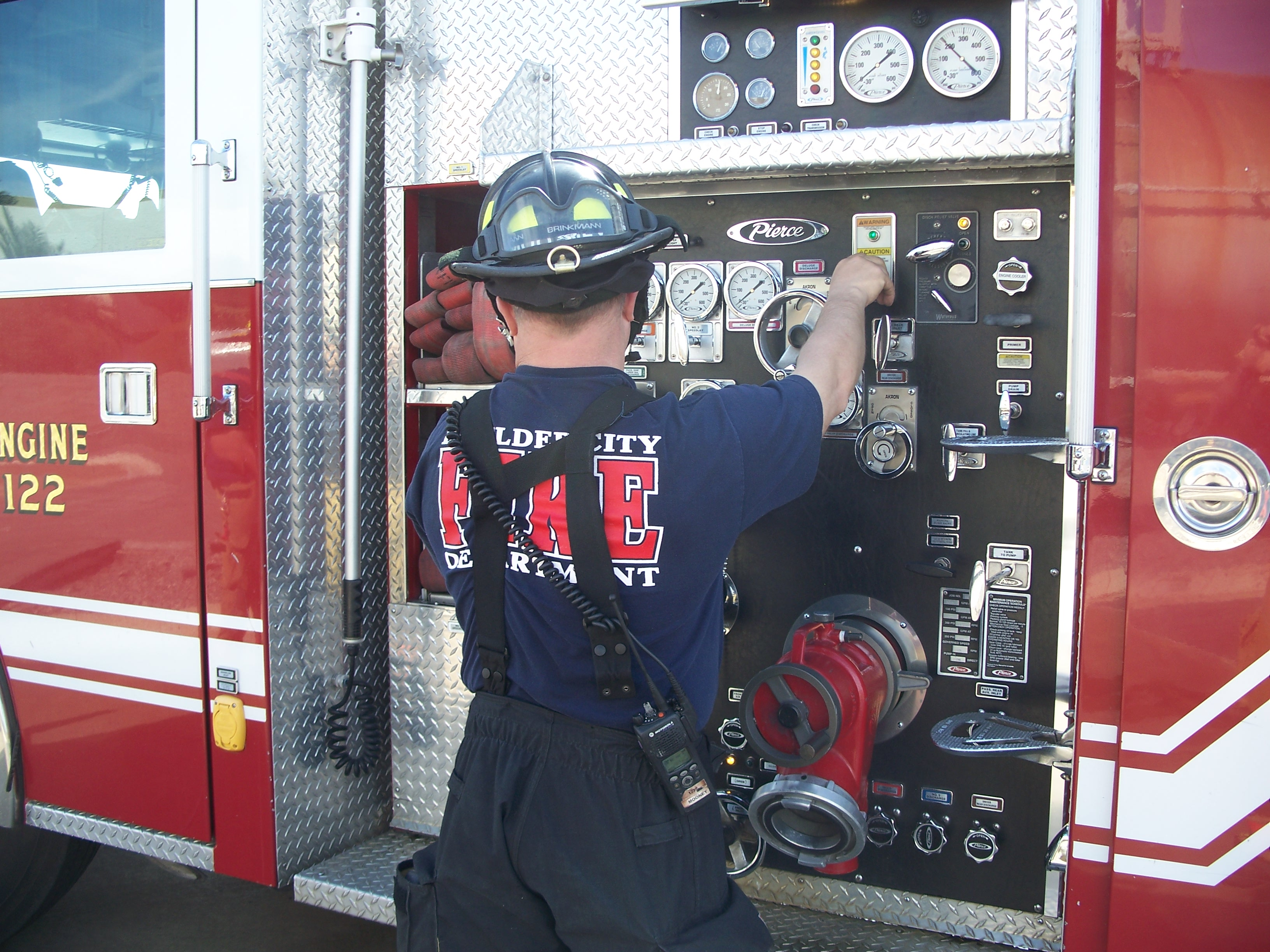Lifestyle

# Types Of Mass Flow Meters And Their Operating PrinciplesPublished on May 28th, 2018

Many chemical and industrial processes require precise delivery of fluids to ensure the composition of the final product is as it should be.

If the quantities of liquids or gases used in the processes is not accurately measured, this may greatly affect the quality of the products and may also jeopardize the health and safety of the people working in the production facility, for instance, in cases where the gases or liquids are highly flammable.The rate of fluid flow can be measured using either a mass flow meter or a volumetric flow meter. Mass flow meters have an advantage over volumetric flow meters because the mass of a fluid is not affected by variations in pressure and temperature while the volume of a fluid is.Therefore, when a volumetric flow meter is used, additional computations have to be made to take into account the pressure and temperature differences. This article focuses on three types of mass flow meters and their operating principles.

## Types of mass flow meters

1. Thermal mass flow meterOne of the most common thermal mass flow meter uses is in low gas flow rates. The heat capacity of the fluid must be known. There are two designs available; inserted probe and heated sample tube.

In the heated sample tube mass flow meter, the tube through which the fluid is flowing is heated and the temperature change measured. The mass of fluid flowing through the tube can then be calculated since the heat capacity of the fluid is known.

The inserted probe mass flow meter works in the same way, but with two probes positioned in the pipe through which the fluid is flowing. The two probes are heated to different temperatures and the rate of heat transfer is measured. The mass flow rate can then be determined since the heat capacity of the fluid is known.

2. The Coriolis mass flow meterThis one is ideal for liquids or very dense gases. The mass of the fluid is measured through inertia. A small actuator is used to vibrate the tube through which the fluid is flowing. A twisting force is produced by the acceleration.

This force is measurable and is proportional to the mass. One huge advantage that this mass flow meter has over the other types, is the fact that the properties of the fluid being measured do not have to be known. That is why these mass flow meters are so widely used today.

3. Differential pressure flow meterAs the name suggests, the principle behind the operation of this flow meter is pressure difference. An obstruction is placed in a tube or pipe through which a fluid is flowing.

The obstruction can be something like a disc but its diameter must be known. The difference in the pressure of the fluid before and after the obstruction is proportional to the volumetric flow rate, the distance between the two reading points, the diameter of the pipe, and the viscosity of the fluid.

Adjustments for pressure and temperature variations are made to produce a standardized mass flow rate.